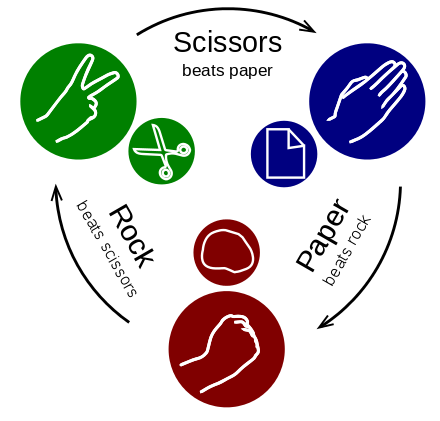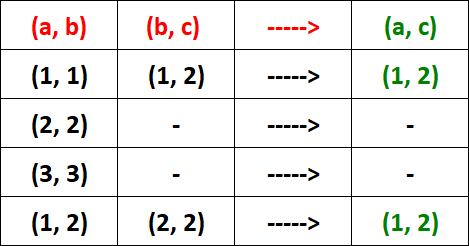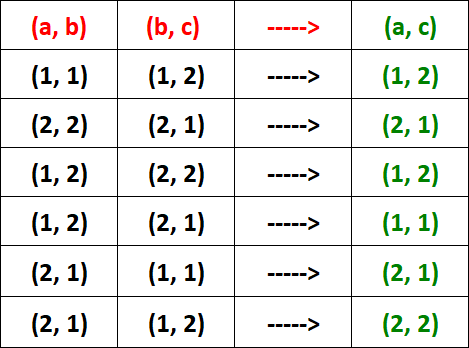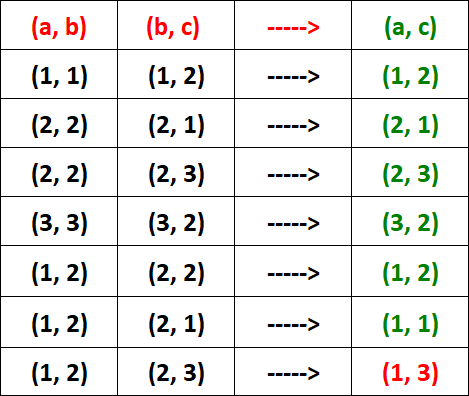Math Concepts

# How to Prove a Relation is Transitive?

16.7k views

 1 Introduction 2 How to tell if a relation is transitive 3 Transitive Relation Example 4 Transitive Questions 5 Summary

14 October  2020

## Introduction

The transitive property comes from the transitive property of equality in mathematics. In math, if A=B and B=C, then A=C. So, if A=5 for instance, then B and C must both also be 5 by the transitive property. This is true in—a foundational property of—math because numbers are constant and both sides of the equals sign must be equal, by definition.Let us take an example of set A as given below to see transitive relations.

A  =  {a, b, c}

Let R be a transitive relation defined on set A.

Then,

R  =  { (a, b), (b, c), (a, c)}

That is,

If ‘a’ is related to ‘b’ and ‘b’ is related to ‘c’, then ‘a’ has to be related to ‘c’.

In simple terms,

a R b, b R c -----> a R c

## How to tell if a relation is transitive?

Now to understand how to prove a relation is transitive, let us understand using common examples.

Compare this concept to the relation of `greater than' for numbers. For instance, if x, y, and z are numbers and we know that x > y and y > z then it must follow that x > z. It follows as x is to the right of y on the number line and y is to the right of z. This relation is called in mathematics and we come to expect it, so when a relation arises that is not transitive, as, in this example, it comes as a surprise. What seems obvious is not always true, so when you think you have a mathematical result you could be wrong. You will always prove a result before you can be sure it is true.

For example, "is greater than," "is at least as great as," and "is equal to" (equality) are transitive relations:

when A > B and B > C, then also A > C

when A ≥ B and B ≥ C, then also A ≥ C

when A = B and B = C, then also A = C.

On the other hand, "is the mother of" is not a transitive relation, because if A is the mother of B, and B is the mother of C, then A is not the mother of C. What is more, it is anti transitive: A can never be the mother of C.Imagine  A be a set in which the relation R defined.

R is said to be transitive, if

(a, b) ∈ R and (b, a) ∈ R ⇒ (a, c) ∈ R,

That means aRb and bRc ⇒ aRc where a, b, c ∈ A.

The relation is said to be non-transitive, if

(a, b) ∈ R and (b, c) ∈ R does not imply (a, c ) ∈ R.

For instance, in the set A of natural numbers if the relation R be defined by ‘x less than y’ then

a < b and b < c implies a < c, that is, aRb and bRc ⇒ aRc.

Hence this relation is transitive.

1. Consider k be given a fixed positive integer.then  R = {(a, a) : a, b  ∈ Z and (a – b) is divisible by k}.

Show that R is a transitive relation.

### Solution:

It is given that  R = {(a, b) : a, b ∈ Z, and (a – b) is divisible by k}.

Assume (a, b) ∈ R and (b, c) ∈ R.
⇒ now  (a – b) is divisible by k and (b – c) is divisible by k.
⇒ again  {(a – b) + (b – c)} is divisible by k.
⇒ (a – c) is divisible by k.
⇒ (a, c) ∈ R.

Hence, (a, b) ∈ R and (b, c) ∈ R   ⇒ (a, c) ∈ R.

So, R is a transitive relation.

2. Consider a relation ρ on the set N is given by “ρ = {(a, b) ∈ N × N: a divisor of b}”. Identify whether ρ is transitive or not transitive relation on set N.

### Solution:

Given ρ = {(a, b) ∈ N × N : a divisor of b}.

Now m, n, p ∈ N and (m, n) ∈ ρ and  (n, p ) ∈ ρ. Then
(m, n) ∈ ρ and  (n, p ) ∈ ρ
⇒ m is a divisor of n and n is a divisor of p
⇒ m is a divisor of p
⇒ (m, p) ∈ ρ

Hence, (m, n) ∈ ρ and (n, p) ∈ ρ ⇒ (m, p) ∈ ρ.

So, R is a transitive relation.

## Transitive Relation Example

To get a better understanding of what is transitive relation so that we can answer “how to tell if a relation is transitive” easily let us go through transitive relation example.

 Example :

Consider A  =  { 1, 2, 3 } and R be a relation defined on  set A as "is less than" and R  = {(1, 2), (2, 3), (1, 3)} Prove transitive.

### Solution :

From the given set A, let
a  =  1
b  =  2
c  =  3

Then, we have

(a, b)  =  (1, 2) -----> 1 is less than 2
(b, c)  =  (2, 3) -----> 2 is less than 3
(a, c)  =  (1, 3) -----> 1 is less than 3

That is, if 1 is less than 2 and 2 is less than 3, then 1 is less than 3.

More clearly,

1R2, 2R3 -----> 1R3

These above points prove that R is a transitive relation.

### Important Note :

For a particular ordered pair in R, if we have (a, b) and we don't have (b, c), then we don't have to check transitive relation for that ordered pair.
So, we have to check transitively, only if we find both (a, b) and (b, c) in R.

A relation R is said to be symmetric if (a,b) € R, (b,c) € R => (a,c)  € R.

 Example

If A is the set of all brothers in a family, then the ”is brother of” relation is transitive over A.
Because if A is the brother of B and B is the brother of C then A is the brother of C.Example

Assume the relation R = {(1,1)(2,2)(3,3)(1,2)(2,3)} is not transitive or intransitive in the set A = {1,2,3} because though (1,2), (2,3) € R , (1,3) is not in R.

## Transitive  Questions

### Problem 1 :

Assume A  =  {1, 2, 3} and R be a relation defined on set A as

R  = {(1, 1), (2, 2), (3, 3), (1, 2)}

Verify R is transitive.

### Solution :

To verify whether R is transitive, we have to see the condition given below for every ordered pair in R.

That is,

(a, b),  (b, c) -----> (a, c)

Let's see the above condition for every ordered pair in R.From the picture above, it is clear that R is transitive.

### Note :

For the two ordered pairs (2, 2) and (3, 3), we don't find the pair (b, c). So, we don't have to check the condition for those ordered pairs.

### Problem 2 :

Consider A  =  {1, 2, 3} and R be a relation defined on set A as

R  = {(1, 1), (2, 2), (1, 2), (2, 1)}

Verify R is a transitive relation.

### Solution :

To verify whether R is a transitive relation, we have to check the condition given below for each ordered pair in R.

That is,

(a, b),  (b, c) -----> (a, c)

Now check the above condition for each ordered pair in R.From the table above, it is clear that R is a transitive relation.

### Problem 3 :

Consider A  =  {1, 2, 3} and R be a relation defined on set A as

R  = {(1, 1), (2, 2), (3, 3), (1, 2), (2, 1), (2, 3), (3, 2)}

Prove relation is transitive.

### Solution :

To verify whether R is a transitive relation, we have to check the condition given below for each and every ordered pair in R.

That is,

(a, b),  (b, c) -----> (a, c)

Please see the above condition for each ordered pair in R.In the table above, for the ordered pair (1, 2), we have both (a, b) and (b, c). But, we don't find (a, c).

Now, we have the ordered pairs (1, 2) and (2, 3) in R. But, we don't have the ordered pair (1, 3) in R.

So, we had to stop the process and conclude that R is not transitive relation or intransitive.

## Summary

Transitivity in mathematics is a property of relationships for which objects of a similar nature may stand to each other. If whenever object A is related to B and object B is related to C, then the relation at that end are transitive relations provided object A is also related to C. Being a child is a transitive relation, being a parent is not.

Transitivity of one relation is so natural that Euclid stated it as the first of his Common Notions

Things which are equal to the same thing are also equal to one another.

In mathematical notations: if A = B and B = C, then certainly A = C. This is a transitive relation! At first glance, this statement lacks content. The reason is of course that the same object may appear in different ways whose identity may not be either obvious or a priori known.

In this blog, we explored transitive relation example, how to tell if a relation is transitive, and transitive relation questions.

Written by Gargi Shrivastava

Related Articles
GIVE YOUR CHILD THE CUEMATH EDGE
Access Personalised Math learning through interactive worksheets, gamified concepts and grade-wise courses